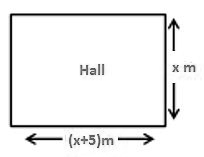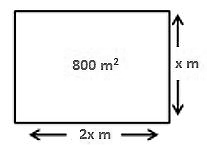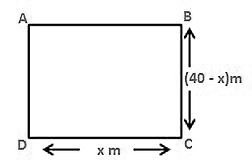# RD Sharma Class 10 Ex 8.11 Solutions Chapter 8 Quadratic Equations

In this chapter, we provide RD Sharma Class 10 Ex 8.11 Solutions Chapter 8 Quadratic Equations for English medium students, Which will very helpful for every student in their exams. Students can download the latest RD Sharma Class 10 Ex 8.11 Solutions Chapter 8 Quadratic Equations pdf, Now you will get step by step solution to each question.

# Chapter 8: Quadratic Equations Exercise – 8.11

### Question: 1The perimeter of the rectangular field is 82 m and its area is 400 m2. Find the breadth of the rectangle?

### Solution:

Let the breadth of the rectangle be (x) m

Given,

Perimeter = 82 m

Area = 400 m2

Perimeter of a rectangle = 2(length + breadth)

80 = 2(length + x)

41 = (length + x)

Length = (41 – x)m

We know,

Area of the rectangle = length * breadth

400 = (41 – x)(x)

400 = 41x – x2

=  x– 41x +400 = 0

= x– 25x – 16x + 400 = 0

= x(x – 25) – 16(x – 25) = 0

= (x – 16)(x – 25) = 0

Either x – 16 = 0 therefore x = 16

Or, x – 25 = 0 therefore x = 25

Hence the breadth of the above mentioned rectangle is either 16 m or 25 m respectively.

### Question: 2The length of the hall is 5 m more than its breadth. If the area of the floor of the hall is 84 m2, what is the length and breadth of the hall?

### Solution:

Le the breadth of the rectangle be x m

Let the length of the hall is 5 m more than its breadth = (x + 5) m

Also given that,

Area of the hall is = 84 m2

The shape of the hall is rectangular

Area of the rectangular hall = length * breadth

84 = x(x + 5)

= x+ 5x – 84 = 0

= x+ 12x – 7x – 84 = 0

= x(x + 12) – 7(x + 12) = 0

= (x + 12)(x – 7) = 0

Either x + 12 = 0 therefore x = – 12

Or, x – 7 = 0 therefore x = 7

Since the value of x cannot be negative

So x = 7

= x + 5 = 12

The length and breadth of the rectangle is 7 and 12 respectively.

### Question: 3

Two squares have sides x and (x + 4) cm. The sum of their area is 656 cm2. Find the sides of the square.

### Solution:

Let S1 and S2 be the two square

Let x cm be the side square S1 and (x + 4) cm be the side of the square S2.

Area of the square S1 = x2 cm2

Area of the square S2 =(x + 4)2 cm2

According to the question,

Area of the square S+ Area of the square S= 656 cm2

= x2 cm+ (x + 4)2 cm= 656 cm2

= x2+ x+ 16 + 8x – 656 = 0

= 2x+ 16 + 8x – 656 = 0

= 2(x+ 4x – 320) = 0

= x+4 x – 320 = 0

= x+ 20x – 16x – 320 = 0

= x(x + 20) – 16(x + 20) = 0

= (x + 20)(x – 16) = 0

Either x + 20 = 0 therefore x = -20

Or, x – 16 = 0 therefore x = 16

Since the value of x cannot be negative so the value of x = 16

The side of the square S1= 16 cm

The side of the square S2 = 20 cm

### Question: 4

The area of the right-angled triangle is 165 cm2. Determine the base and altitude if the latter exceeds the former by 7m.

### Solution:

Let the altitude of the right angles triangle be denoted by x mGiven that the altitude exceeds the base by 7m = x – 7m

We know

Area of the triangle = 1/2 × base × altitude

= 165 = 1/2 × (x − 7) × x

= x(x – 7) = 330

= x– 7x – 330 = 0

= x– 22x + 15x – 330 = 0

= x(x – 22) + 15(x – 22) = 0

= (x – 22)(x + 15) = 0

Either x – 22 = 0 therefore x = 22

Or, x + 15 = 0 therefore x = -15

Since the value of x cannot be negative so the value of x = 22

= x – 7 = 15

The base and altitude of the right angled triangle are 15 cm and 22 cm respectively.

### Question: 5

Is it possible to design a rectangular mango grove whose length is twice its breadth and area is 800 m2.find its length and breadth.

### Solution:

Let the breadth of the rectangular mango grove be x mGiven that length of rectangle is twice of its breadth

Length = 2x

Area of the grove = 800 m2

We know,

Area of the rectangle = length * breadth

= 800 = x(2x)

= 2x– 800 = 0

= x– 400 = 0

Breadth of the rectangular groove is 20 m

Length of the rectangular groove is 40 m

Yes, it is possible to design a rectangular groove whose length is twice of its breadth.

### Question: 6

Is it possible to design a rectangular park of perimeter 80 m and area 400 m2?. If so find its length and breadth.

### Solution:

In order to prove the given condition let us assume that the length of the rectangular park is denoted by x mGiven that,

Perimeter = 8 cm

Area = 400 cm2

Perimeter of the rectangle = 2(length + breadth)

We know,

Area of the rectangle = (length) (breadth)

= 400 = x(40 – x)

= 40x – x= 400

= x– 40x + 400 = 0

= x– 20x – 20x + 400 = 0

= x(x – 20) – 20(x – 20) = 0

= (x – 20)(x – 20) = 0

= (x – 20)2 = 0

= x – 20 = 0 therefore x =20

Length of the rectangular park is = 20 m

Breadth of the rectangular park = (40 – x) = 20 m

Yes, it is possible to design a rectangular Park of perimeter 80 m and area 400 m2

### Question: 7

Sum of the area of the square is 640 m2.if the difference of their perimeter is 64 m, find the sides of the two squares.

### Solution:

Let the two squares be S1 and Srespectively.

Let he sides of the square Sbe x m and the sides of the square Sbe y m

Given that the difference of their perimeter is 64 m

We know that the

Perimeter of the square = 4(side)

Perimeter of the square S1 = 4x m

Perimeter of the square S2 = 4y m

Now, difference of their perimeter is 64 m

= 4x – 4y = 64

x – y = 16

x = y + 16

Also, given that the sum of their two areas

= area of the square 1 +area of the square 2

= 640 = x+ y2

= 640 = (y + 16)+ y2

= 2y+ 32y + 256 – 640 = 0

= 2y+ 32y – 384 = 0

= 2(y+ 16y – 192) = 0

= y+ 16y – 192 = 0

= y+ 24y – 8y – 192 = 0

= y(y + 24) – 8(y + 24) = 0

= (y + 24)(y – 8) = 0

Either y + 24 = 0 therefore y = -24

Or, y – 8 = 0 therefore y = 8

Since the value of y cannot be negative so y = 8

Side of the square 1 = 8 m

Side of the square 2 = 8 + 16 = 24 m

The sides of the squares 1 and 2 are 8 and 24 respectively.

All Chapter RD Sharma Solutions For Class10 Maths

I think you got complete solutions for this chapter. If You have any queries regarding this chapter, please comment on the below section our subject teacher will answer you. We tried our best to give complete solutions so you got good